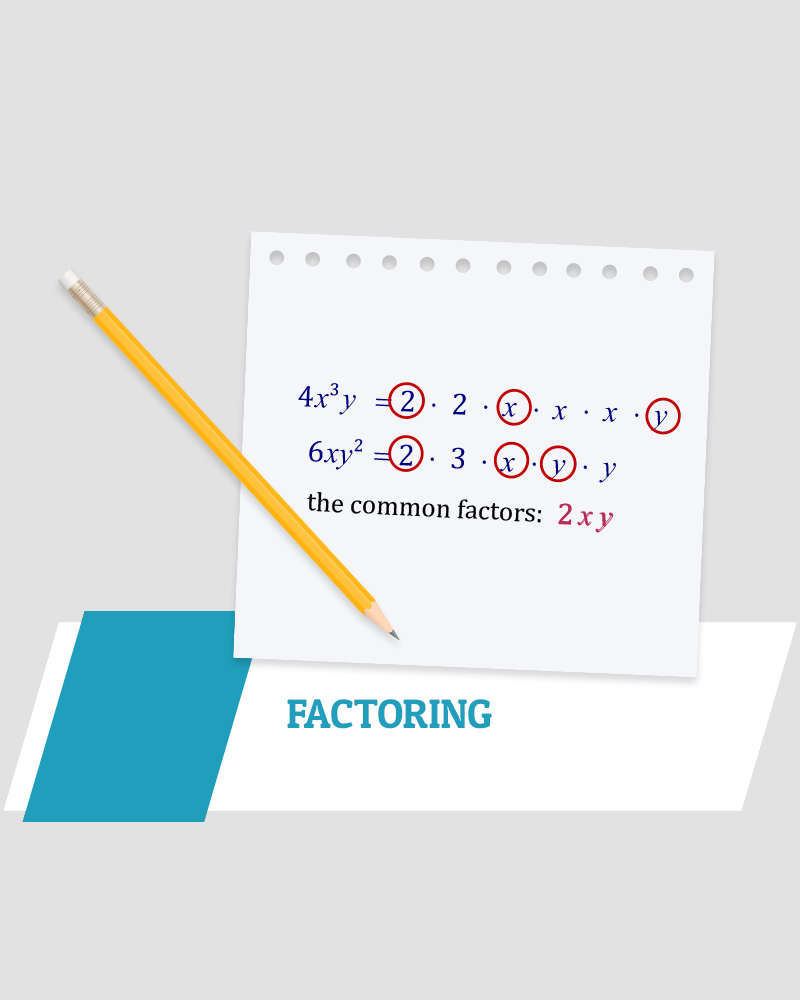# Factoring

In this video,  I ‘d like to show some examples of factoring method.

First, always check to see if there are common factors in any type of expressions.   I showed three types of formulas in this video,difference of two squares, quadratic trinomials, and perfect square trinomials.

However, we can use the cross method for all three types questions using the quadratic trinomials as the standard one.

Difference of two squares formula has the middle term 0, and Perfect square trinomials formula has 2 equal middle terms.

In conclusion, if you can notice each formula, then it is efficient and quicker method, but even you do not recognize each formula, the cross method works for all three types.